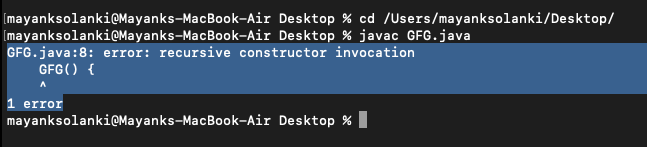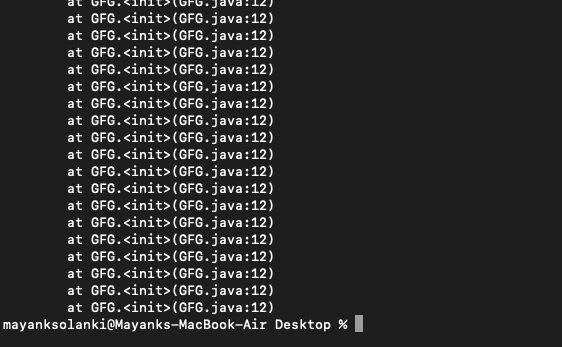Open in App
Not now

# Recursive Constructor Invocation in Java

• Last Updated : 26 Sep, 2021

The process in which a function calls itself directly or indirectly is called recursion and the corresponding function is called a recursive function. In the recursive program, the solution to the base case is provided and the solution of the bigger problem is expressed in terms of smaller problems. Here recursive constructor invocation and stack overflow error in java. It is as shown below in the example as follows:

Example

## Java

 `// Java Program to Illustrate Recursion` `// Main class``public` `class` `GFG {` `    ``static` `int` `count = ``0``;` `    ``// Method 1``    ``// Recursive method``    ``static` `void` `function()``    ``{``        ``count = count + ``1``;``        ``if` `(count <= ``5``) {` `            ``System.out.println(``"Call "` `+ count);``            ``function();``        ``}``    ``}` `    ``// Method 2``    ``// Main driver method``    ``public` `static` `void` `main(String[] args) { function(); }``}`

Output

```Call 1
Call 2
Call 3
Call 4
Call 5```

Recursive Constructor Invocation

If a constructor calls itself, then the error message “recursive constructor invocation” occurs. The following program is not allowed by the compiler because inside the constructor we tried to call the same constructor. The compiler detects it instantly and throws an error.

Example:

## Java

 `// Java program to Illustrate How Recursive``// Constructor Invocation Error is Occurred` `// Main class``class` `GFG {` `    ``// Constructor of this class``    ``// Inside we are trying to call the same constructor``    ``GFG()``    ``{``        ``// This keyword refers to same instance itself``        ``this``();``    ``}` `    ``// Main driver method``    ``public` `static` `void` `main(String[] args)``    ``{` `        ``// Creating an object of class inside main()``        ``GFG obj = ``new` `GFG();``    ``}``}`

Output:Now let us discuss what exactly we do refer to by Stack overflow error and why does it occur. Stack overflow error occurs if we do not provide the proper terminating condition to our recursive function or template, which means it will turn into an infinite loop.

Implementation:

Here we have created a GFG object inside the constructor which is initialized by calling the constructor, which then creates another GFG object which is again initialized by calling the constructor and it goes on until the stack overflows. This can be justified from the illustration as follows:

Example:

## Java

 `// Java program to Illustrate Stack Overflow Error` `// Main class``public` `class` `GFG {` `    ``// Constructor of this class``    ``GFG()``    ``{` `        ``// Creating an object of GFG class inside the``        ``// constructor which is initialized by calling the``        ``// constructor, which is initialized by``        ``// calling the constructor and it goes on``        ``GFG obj1 = ``new` `GFG();``    ``}` `    ``// Main driver method``    ``public` `static` `void` `main(String[] args)``    ``{` `        ``// Creating an object of this class``        ``GFG obj = ``new` `GFG();``    ``}``}`

Output:My Personal Notes arrow_drop_up